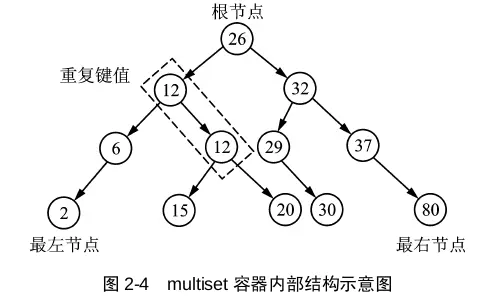# 480. Sliding Window Median 滑动窗口中位数

@TOC

## # 题目描述

Median is the middle value in an ordered integer list. If the size of the list is even, there is no middle value. So the median is the mean of the two middle value.

Examples:

`[2,3,4]` , the median is `3`

`[2,3]`, the median is `(2 + 3) / 2 = 2.5`

Given an array nums, there is a sliding window of size k which is moving from the very left of the array to the very right. You can only see the k numbers in the window. Each time the sliding window moves right by one position. Your job is to output the median array for each window in the original array.

For example,

``````Given nums = [1,3,-1,-3,5,3,6,7], and k = 3.

Window position                Median
---------------               -----
[1  3  -1] -3  5  3  6  7       1
1 [3  -1  -3] 5  3  6  7       -1
1  3 [-1  -3  5] 3  6  7       -1
1  3  -1 [-3  5  3] 6  7       3
1  3  -1  -3 [5  3  6] 7       5
1  3  -1  -3  5 [3  6  7]      6
Therefore, return the median sliding window as [1,-1,-1,3,5,6].
``````

Note:

1. You may assume k is always valid, ie: k is always smaller than input array's size for non-empty array.

## # 解题方法

### # MultiSet 遍历到中位数位置

1. 在 C++ 中 `set/multiset` 是有序的集合，它们是基于红黑树实现的。其中 `set` 会对元素去重，而 `multiset` 可以有重复元素。

2. 在 Java 中 `TreeSet` 是有序的集合，它也是基于红黑树实现的。 `TreeSet` 会对元素去重。

3. 在 Python 中 `heapq` 实现了堆的算法，它不会对元素去重。1. 首先定义了结果数组 res 和 multiset；
2. 遍历输入中的每个元素；
3. 如果 multiset 中的元素超过了 k 个，需要把滑动窗口最左边 i - k 位置元素从 multiset 中删除（multiset 自动维护有序性）；
4. 把遍历到的当前元素插入到 multiset 中（multiset 自动维护有序性）；
5. 如果当前的位置达到了下标 k - 1，说明滑动窗口中有 k 个元素，开始求滑动窗口的中位数。

C++ 代码如下：

``````class Solution {
public:
vector<double> medianSlidingWindow(vector<int>& nums, int k) {
vector<double> res;
multiset<double> st;
for (int i = 0; i < nums.size(); ++i) {
if (st.size() >= k) st.erase(st.find(nums[i - k]));
st.insert(nums[i]);
if (i >= k - 1) {
auto mid = st.begin();
res.push_back((*mid + *prev(mid, (1 - k % 2))) / 2);
}
}
return res;
}
};
``````

### # MultiSet维护中位数指针

C++代码如下，时间复杂度是O(N)，明显比上面的做法要快。

``````class Solution {
public:
vector<double> medianSlidingWindow(vector<int>& nums, int k) {
vector<double> res;
multiset<double> st(nums.begin(), nums.begin() + k);
auto mid = next(st.begin(), k / 2);
for (int i = k; ;++i) {
res.push_back((*mid + *prev(mid, 1 - k % 2)) / 2);
if (i == nums.size()) return res;
st.insert(nums[i]);
if (nums[i] < *mid) --mid;
if (nums[i - k] <= *mid) ++mid;
st.erase(st.lower_bound(nums[i - k]));
}
return res;
}
};
``````

## # 日期

2019 年 9 月 14 日 —— 假期的生活就是不规律 2021 年 2 月 3 日 —— 坚持日更公众号10天了！